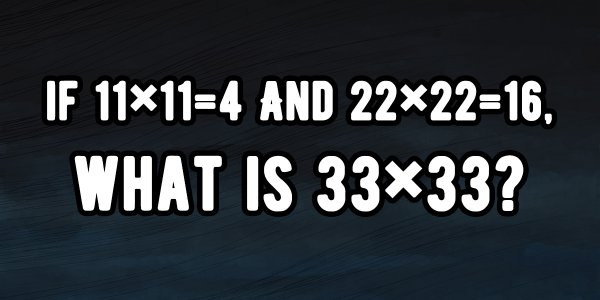# If 11×11=4 And 22×22=16, What Is 33×33?A simple puzzle shared on Quora is going viral- do you know the answer?

The puzzle was shared by user- Ismael Nunez a student at Perth Amboy High School in which she said:

Well, there are multiple potential patterns but it is most likely this:

11×11=4

But the 11 would be an addition of 1 and 1 instead. So:

(1+1)∗(1+1)=4

2×2=4

4=4

The same rule would apply to 22 x 22 = 16, where the 22 becomes the addition of 2 and 2:

(2+2)∗(2+2)=16

4×4=16

16=16

So now we apply the rule to 33×33:

33×33=?

(3+3)∗(3+3)=?

6×6=36

?=36

Hence the answer will be 36.